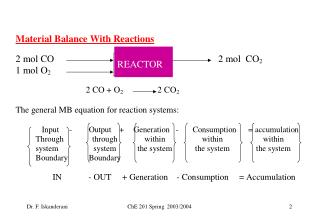DownloadDownload PresentationREACTOR

# REACTOR

Télécharger la présentation## REACTOR

- - - - - - - - - - - - - - - - - - - - - - - - - - - E N D - - - - - - - - - - - - - - - - - - - - - - - - - - -
##### Presentation Transcript

1. REACTOR ChE 201 Spring 2003/2004

2. Q : Is total moles in = total moles out ? Q : Is total mass in = total mass out ? Mass in mass out 2x28 + 32 = 2x44 88 = 88 ChE 201 Spring 2003/2004

3. Let us now look at the elements (atoms) C and O: ChE 201 Spring 2003/2004

4. Consumption of CH4 = 10 Example 2 (Note : All calculations are in moles) • 10 moles of CH4 are burned completely with 80 moles of O2. Find all components out. • CH4 + 2O2 CO2 + 2H2O • accu = in - out + generation - consumption • CH40 = 10 - 0 + 0 - consumption ChE 201 Spring 2003/2004

5. CH4 + 2O2 CO2 + 2H2O • accu = in - out + generation - consumption • O2 0 = 80 - ? + 0 - 10 x 2/1 O2 OUT = 80 - 20 = 60 ChE 201 Spring 2003/2004

6. CH4 + 2O2 CO2 + 2H2O • accu = in - out + generation - consumption • CO20 = 0 - ? + - 0 10 x 1/1 CO2 OUT = 10 ChE 201 Spring 2003/2004

7. CH4 + 2O2 CO2 + 2H2O • accu = in - out + generation - consumption • H2O 0 = 0 - ? + - 0 10 x 2/1 H2O OUT = 20 ChE 201 Spring 2003/2004

8. SUMMARY • CH4 + 2O2 CO2 + 2H2O • In 10 80 • Consumed 10 20 • Generated 0 0 10 20 . • Out 0 60 10 20 ChE 201 Spring 2003/2004

9. (Now Solve using ATOMIC BALANCES) • IN OUT • CH4 O2 CO2 H2O O2 • IN = OUT • C bal 10 + 0 = + 0 + 0 1 x nCO2 or x1 Moles C =1x moles of CO2 THEREFORE nCO2 OUT = 10 ChE 201 Spring 2003/2004

10. ATOMIC BALANCES • IN OUT • CH4 O2 CO2 H2O O2 • IN = OUT • H bal 4x10 + 0 = 0 +2nH2O + 0 Thus nH2O= 40/2 = 20 moles ChE 201 Spring 2003/2004

11. ATOMIC BALANCES 80 moles O2 • IN OUT • CH4 O2 CO2 H2O O2 • IN = OUT • O bal 0 + 2x80 = 2 x nCO2 +1nH2O + 2nO2,out Moles O =2x moles of CO2 Moles O =1x moles of H2O ChE 201 Spring 2003/2004

12. 80 moles O2 • IN OUT • CH4 O2 CO2 H2O O2 • IN = OUT • O bal 0 + 2x80 = 2 x nCO2 +1nH2O + 2nO2,out Moles O =2x moles of CO2 Moles O =1x moles of H2O Thus, nO2,out = (160 - 20 - 20)/2 =60moles ChE 201 Spring 2003/2004

13. FOR REACTION PROBLEMS , with no accuulation, the following is true: 1. total mass in = total mass out 2. for every element (atom) present in the problem, Mass of element in = Mass of element out Moles of element in = Moles of element out 3. Anyinert compoundsthat are presentand not involved in the reaction, component balances can be carried out for them ChE 201 Spring 2003/2004